Math Central - mathcentral.uregina.caMathematics with a Human Face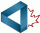sponsored by the Canadian Mathematical Society
math with a human face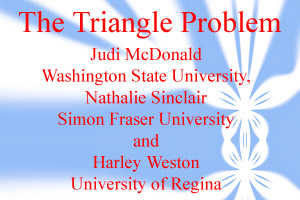On this page we describe an unsolved problem in geometry dealing with reflected triangles. [This problem was solved in 2012 by Grégoire Nicollier. A complete solution is in his paper that appears in Forum Geometricorum.] This problem led to the above graphic, which is also the background image on the Mathematicians at Work poster. When Judi and Harley were looking for a background for the poster, Nathalie introduced them to this problem and the algorithm that produced the graphic.

Start with triangle ABC and reflect each vertex across the opposite side; you get triangle A1B1C1— the first reflected triangle.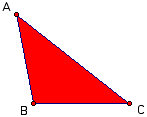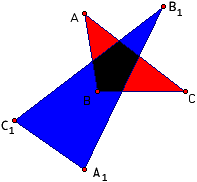What is the relationship between the triangles ABC and A1B1C1? When ABC is isosceles, so is A1B1C1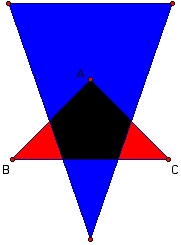and when ABC is equilateral, so is A1B1C1. You might want to verify these two statements by yourself. Suppose now that we fix the position of B and C, but move A to change the shape of the original triangle ABC. Then the shape of A1B1C1 changes also. If your browser has java enabled you can open a new window that allows you to move A, B or C and observe how the reflected triangle changes.

Of the two triangles ABC and A1B1C1, it is often the second that looks more like an equilateral triangle. To get a sense of “how close to being equilateral” it is, we need a measure. There are many measures we can use: we could measure how far the angles are from being 60 degrees, or how far the lengths of the sides are from being equal. The measure we are going to use is the ratio of the perimeter squared to the area. This ratio is at a minimum for an equilateral triangle (see, for example, Kazarinoff). The minimum is 12sqrt(3), which is approximately 20.7.

In addition to looking at the triangle A1B1C1 generated by reflecting the vertices of ABC, we want to reflect the vertices of A1B1C1 to generate a second reflected triangle A2B2C2. Then using the vertices of A2B2C2 we generate a third reflected triangle A3B3C3, then a fourth, and so on. We call these the first, second, third and fourth iterations of the reflection process. Here are some pictures of three iterations starting with A in different positions. Again, if your browser has java enabled you can open a new window that allows you to move A and observe how the reflected triangles change.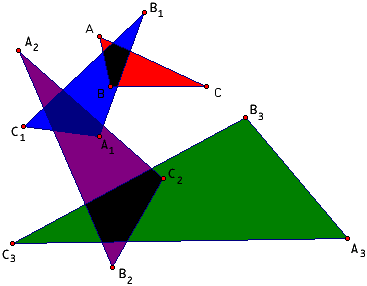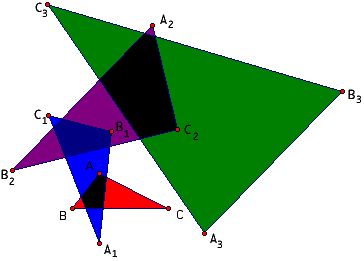Originally it was conjectured that whatever the triangle ABC, the sequence of triangles A1B1C1, A2B2C2, A3B3C3,… would always approach an equilateral triangle (Schwartz). As is usually the case with mathematical research, people began looking for either a proof of the conjecture or a counterexample to the conjecture. Keith Dean showed that a triangle with angles in the ratios of 1:2:4 (that with angles that measure 25 5/7, 51 3/7 and 102 6/7 degrees respectively) reflects into another triangle with the same angles, and hence repeated reflections will never produce an equilateral triangle. Thus the conjecture is false. Patrick Callaghan also found a characterization of the triangles that will map onto a straight line. For these triangles also, the conjecture is false.

Thus the problem is:

For what triangles ABC does the sequence of iterated triangles approach an equilateral triangle?

Nathalie devised a procedure for “seeing” many cases at once. This is the procedure used to produce the background graphic on the Mathematicians at Work poster.

Fix the base of the triangle BC, chose another point A in the plane to form the triangle ABC, perform the reflection twice to produce A2B2C2 and then colour the point A according to how close A2B2C2 is to being equilateral. A is painted blue if A2B2C2 is close to being equilateral and white if A2B2C2 is far from being equilateral. This procedure is repeated for all points A in a rectangular region in the plane to generate the graphic below.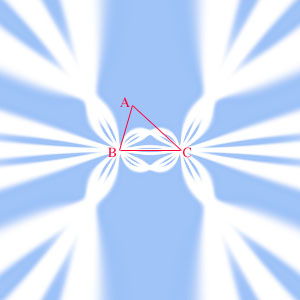Two iterations1

There are large regions in the graphic that are coloured blue. If A is in one of these regions then A2B2C2 is close to being equilateral. There are, however, regions coloured white. If A is in one of these regions then A2B2C2 is far from being equilateral.

If the reflection is iterated three times to produce A3B3C3 and A is coloured according to how close A3B3C3 is to being equilateral, then the resulting image below is similar to that above, but with a more complex pattern in the white regions.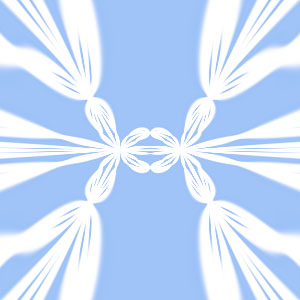Three iterations1

Similarly, the graphic below, which was produced by four iterations, has even more blue and less white. We can infer from this that as we increase the number of iterations, more of the initial triangles have a final reflected triangle that is close to being equilateral.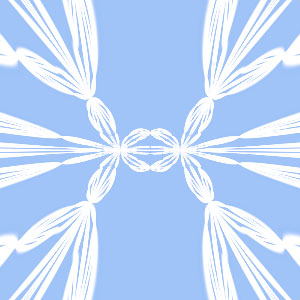Four iterations1

At this stage, we can reflect some more on what we are seeing. If we do enough iterations, will the entire picture be blue? It shouldn't, we know that there are some triangles (described above) that do not converge to equilateral triangles. Regions corresponding to these triangles should stay white. You can use the java applets above to discover some positions for A that seem to result in triangles that converge to a line. Why are there other regions that are white? Can you find other conditions/positions for A that will result in a sequence of triangles that doesn't converge to an equilateral triangle? Do these images help in addressing the original question; for what triangles ABC does the sequence of iterated triangles approach an equilateral triangle? Computer graphics can give insight into answering these questions but a complete answer requires a mathematical proof.

If you find something interesting in your explorations of these questions, please let us know at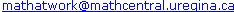. Maybe your name can be added to the list of people who have made contributions towards a solution of this problem.

In August of 2006 we received this note from Pasquale Nardone, Université Libre de Bruxelles.

1 Graphics produced by Matthew McKague

References

Judah Schwartz, "Can technology help us make the mathematics curriculum intellectually stimulating and socially responsible?" International Journal of Computers for Mathematical Learning, 4,1999, pages 99-119.

Nicholas D. Kazarinoff, Geometric Inequalities, Random House, New York, 1961.
 Math Central is supported by the University of Regina and the Pacific Institute for the Mathematical Sciences.about math central :: site map :: links :: notre site français